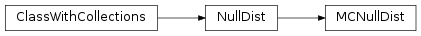# mvpa2.clfs.stats.MCNullDist¶class `mvpa2.clfs.stats.``MCNullDist`(permutator, dist_class=<class 'mvpa2.clfs.stats.Nonparametric'>, measure=None, **kwargs)

Null-hypothesis distribution is estimated from randomly permuted data labels.

The distribution is estimated by calling fit() with an appropriate `Measure` or `TransferError` instance and a training and a validation dataset (in case of a `TransferError`). For a customizable amount of cycles the training data labels are permuted and the corresponding measure computed. In case of a `TransferError` this is the error when predicting the correct labels of the validation dataset.

The distribution can be queried using the `cdf()` method, which can be configured to report probabilities/frequencies from `left` or `right` tail, i.e. fraction of the distribution that is lower or larger than some critical value.

This class also supports `FeaturewiseMeasure`. In that case `cdf()` returns an array of featurewise probabilities/frequencies.

Notes

Available conditional attributes:

• `dist_samples`: Samples obtained for each permutation
• `skipped+`: # of the samples which were skipped because measure has failed to evaluated at them

(Conditional attributes enabled by default suffixed with `+`)

Methods

 `cdf`(x) `clean`() Clean stored distributions `dists`() `fit`(measure, ds) Fit the distribution by performing multiple cycles which repeatedly permuted labels in the training dataset. `p`(x[, return_tails]) Returns the p-value for values of `x`. `rcdf`(x)

Initialize Monte-Carlo Permutation Null-hypothesis testing

Parameters: permutator : Node Node instance that generates permuted datasets. dist_class : class This can be any class which provides parameters estimate using `fit()` method to initialize the instance, and provides `cdf(x)` method for estimating value of x in CDF. All distributions from SciPy’s ‘stats’ module can be used. measure : Measure or None Optional measure that is used to compute results on permuted data. If None, a measure needs to be passed to `fit()`. enable_ca : None or list of str Names of the conditional attributes which should be enabled in addition to the default ones disable_ca : None or list of str Names of the conditional attributes which should be disabled tail : {‘left’, ‘right’, ‘any’, ‘both’} Which tail of the distribution to report. For ‘any’ and ‘both’ it chooses the tail it belongs to based on the comparison to p=0.5. In the case of ‘any’ significance is taken like in a one-tailed test. descr : str Description of the instance

Methods

 `cdf`(x) `clean`() Clean stored distributions `dists`() `fit`(measure, ds) Fit the distribution by performing multiple cycles which repeatedly permuted labels in the training dataset. `p`(x[, return_tails]) Returns the p-value for values of `x`. `rcdf`(x)
`cdf`(x)
`clean`()

Clean stored distributions

Storing all of the distributions might be too expensive (e.g. in case of Nonparametric), and the scope of the object might be too broad to wait for it to be destroyed. Clean would bind dist_samples to empty list to let gc revoke the memory.

`dists`()
`fit`(measure, ds)

Fit the distribution by performing multiple cycles which repeatedly permuted labels in the training dataset.

Parameters: measure: Measure or None : A measure used to compute the results from shuffled data. Can be None if a measure instance has been provided to the constructor. ds: `Dataset` which gets permuted and used to compute the : measure/transfer error multiple times.
`rcdf`(x)